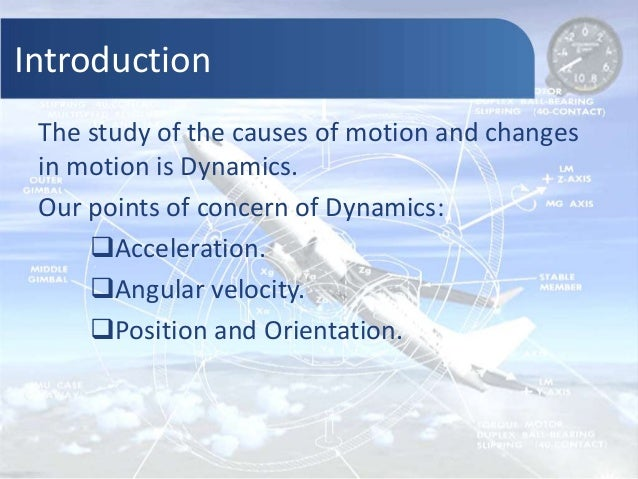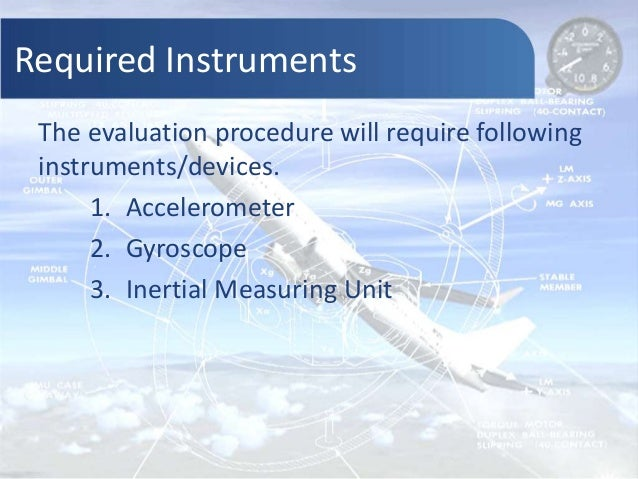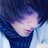Successfully reported this slideshow.Upcoming SlideShare
×

ofUpcoming SlideShare
Better motion control using accelerometer/gyroscope sensor fusion
Next

4

Share

# Evaluation of dynamics | Gyroscope, Accelerometer, Inertia Measuring Unit and Kalman Filter

Robo India presents theory and working principles of Inertia Measuring unit (IMU), gyroscope, accelerometer and Kalman Filter. It is an important controlling part of unmanned Arial vehicles (UAV)

We have named it as evaluation of dynamics.

We welcome all of your views and queries, we are found at-
website: http://roboindia.com
mail- info@roboindia.com

See all

See all

### Evaluation of dynamics | Gyroscope, Accelerometer, Inertia Measuring Unit and Kalman Filter

1. 1. Evaluation of Dynamics Robo India www.roboindia.com
2. 2. Introduction The study of the causes of motion and changes in motion is Dynamics. Our points of concern of Dynamics: Acceleration. Angular velocity. Position and Orientation.
3. 3. Required Instruments The evaluation procedure will require following instruments/devices. 1. Accelerometer 2. Gyroscope 3. Inertial Measuring Unit
4. 4. Accelerometer | Introduction Accelerometer is a device that measures acceleration with respect to gravity free space reference (free fall reference). A typical concept of accelerometer
5. 5. Draw backs of typical accelerometer • It would be more complex for multiple axis. • Measurement would be more complex. • Difficult to fit in applications. • Maintenance would be high. Micro Electro Mechanical System (MEMS) accelerometer sensors overcome all such limitations.
6. 6. MEMS Accelerometer MEMS accelerometer are very tiny electromechanical sensors that gives output in electronic form(i.e. voltage/current) . Types of MEMS accelerometers: • Capacitive • Piezoelectric • Piezoresistive etc.
7. 7. Capacitive Accelerometer In capacitive accelerometer micro-machined silicon material is used. The distance between these materials varies with the acceleration faced by the accelerometer that causes to change the capacitance which leads to change output voltage. The particular output voltage defines a particular acceleration. Out put can be: 1. Analogue 2. Digital
8. 8. Useful Terminologies • Output: accelerometer sensor gives voltage output that indicates the acceleration faced by the sensor in terms of g (acceleration due to gravity (g) = 9.81 m/sec2). • Sensitivity: The rate of change in output voltage with the acceleration. (Unit: mV / g) • Ratiometricity: It means a linear relation between output voltage and sensitivity with respect to change in applied supply voltage. • Zero g (0g): standard output voltage that represents a gravity free space, means the accelerometer is facing no acceleration.
9. 9. Capacitive Accelerometer | Concept
10. 10. Acceleration Measurement
11. 11. Measurement of angle & acceleration R = √ ( Accx 2 + Accy 2 + Accz 2) Ax = cos-1(Accx / R) Ay = cos-1(Accy / R) Az = cos-1(Accz / R) Where: Vx, Vy, Vz : Output for respective axis ACC x, ACCy, ACCz : Acceleraton of respective axis R : Resultant Vector A x, Ay, Az : Angle about respective axis
12. 12. Application of Accelerometers • Engineering: to detect acceleration, engine testing, air bag operations, breaking system and various industrial applications. • Robotics: Motion sensing, hand held devices to save battery consumption. • 3D-Gamming: Tilt & motion sensor • Cell phones / PDA : Screen orientation, gamming sensor, image stability • Navigation and dead reckoning: e-compass • Laptop PCs, HDD audio and video player: Free fall detects to prevent data damaging in Hard Disk Drives (HDD).
13. 13. Gyroscope Gyroscope is a device that resists to change its position, its position doesn’t change with the changes occurs in external surroundings. This property helps extremely in determining the angular velocity for the balancing, landing, turning and taking off of air-vehicles.
14. 14. MEMS Gyroscope Electronic(MEMS) gyroscope sensors are different from the traditional and mechanical gyroscopes. A very tiny IC (Plastic Land Grid Array, LGA package) does the same work and gives output in the desired manner, which makes the electronic gyroscope very easy to use in automation. MEMS Gyroscope works on the Coriolis force concept. A driving mass is kept in continuous oscillating movement, the driving mass acts as sensing element and reacts to the applied angular velocity. The whole arrangement is made up by the integration of actuators and accelerometers on a micro-machined chip.
15. 15. Measuring Gyro output Angular velocities: Ωx = (Vx-V0)/Sensitivity 0/sec Ωy = (Vy-V0)/Sensitivity 0/sec Ωz = (Vz-V0)/Sensitivity 0/sec Where: Vx , Vy & Vz :Output voltages for X,Y and Z axis. Ωx , Ωy & Ωz :Angular velocities about X, Y & Z axis x, 𝒙′ : new and old position 𝑻 − 𝑻′ : Time difference Position: 𝒙 = 𝒙′ + 𝜴 (𝑻 − 𝑻′)
16. 16. Application of Gyroscope • Spaceship • Ship / and motor boats • Airplanes • Unmanned air vehicles • Racing cars • Gamming consoles • Robotics • Dead reckoning (vehicle navigation) • Motion sensing in mobile phones and PDA’s
17. 17. Inertial Measuring Unit (IMU) Inertial Measuring Units are the integration of Accelerometers and Gyroscopes that measures velocity, orientation, acceleration, angular velocity and gravitational force. • Why IMU? • Advantages of IMU • Requirement of filter
18. 18. Kalman Filter Kalman Filter was proposed by Hungarian-American engineer Rudolf Emil Kalman. Kalman Filter is a mathematical estimation algorithm that involves a wide range of processes and applications. The Kalman Filter has main function of combining the measurement of time series data of the same variables but from the different sensors and to forecast the state. It predicts the state and corrects it.
19. 19. Kalman Filter
20. 20. ROBO INDIA
•Apr. 20, 2019
•#### rashmimordia

Mar. 17, 2017
•#### wren4uonline

Jun. 18, 2015
•#### HarrisDams83

May. 11, 2015

Total views

2,792

On Slideshare

0

From embeds

0

Number of embeds

10

143

Shares

0